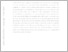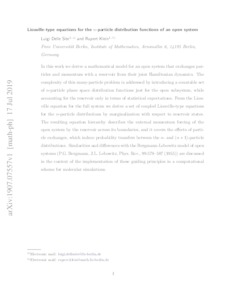Repository: Freie Universität Berlin, Math Department

# Liouville-type equations for the n-particle distribution functions of an open system

Delle Site, L. and Klein, R. (2020) Liouville-type equations for the n-particle distribution functions of an open system. Journal of Mathematical Physics, 61 (8).Preview

804kB

Official URL: https://doi.org/10.1063/5.0008262

## Abstract

In this work we derive a mathematical model for an open system that exchanges particles and momentum with a reservoir from their joint Hamiltonian dynamics. The complexity of this many-particle problem is addressed by introducing a countable set of n-particle phase space distribution functions just for the open subsystem, while accounting for the reservoir only in terms of statistical expectations. From the Liouville equation for the full system we derive a set of coupled Liouville-type equations for the n-particle distributions by marginalization with respect to reservoir states. The resulting equation hierarchy describes the external momentum forcing of the open system by the reservoir across its boundaries, and it covers the effects of particle exchanges, which induce probability transfers between the n- and (n+1)-particle distributions. Similarities and differences with the Bergmann-Lebowitz model of open systems (P.G.Bergmann, J.L. Lebowitz, Phys.Rev., 99:578--587 (1955)) are discussed in the context of the implementation of these guiding principles in a computational scheme for molecular simulations.

Item Type: Article SFB 1114 Preprint in arXiv:1907.07557 article reference: 083102 Mathematical and Computer Sciences > Mathematics > Applied Mathematics Department of Mathematics and Computer Science > Institute of Mathematics > Geophysical Fluid Dynamics Group 2363 Silvia Hoemke 08 Aug 2019 10:23 10 Aug 2020 09:55

Repository Staff Only: item control page